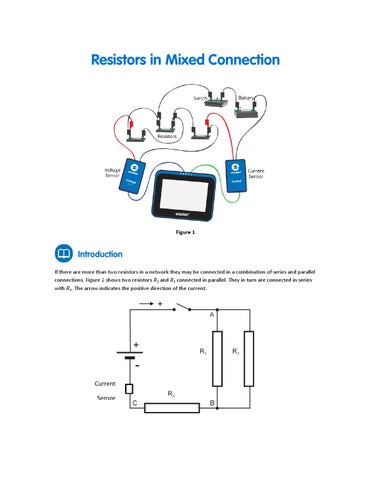Figure 1

If there are more than two resistors in a network they may be connected in a combination of series and parallel connections. Figure 2 shows two resistors R1 and R2 connected in parallel. They in turn are connected in series with R3. The arrow indicates the positive direction of the current.

Current Sensor

Figure 2 To calculate the equivalent resistance we must first calculate the equivalent resistance of the two resistors in parallel, R1 and R2: 1 1 1 = + (1) đ?&#x2018;&#x2026;1,2 đ?&#x2018;&#x2026;1 đ?&#x2018;&#x2026;2 Then add this to R3 to get the total equivalent resistance of the circuit: đ?&#x2018;&#x2026;đ??¸đ?&#x2018;&#x201E;đ?&#x2018;&#x2C6;đ??źđ?&#x2018;&#x2030;đ??´đ??żđ??¸đ?&#x2018; đ?&#x2018;&#x2021; = đ?&#x2018;&#x2026;1,2 + đ?&#x2018;&#x2026;3

(2)

einsteinâ&#x201E;˘Tablet with MiLAB or Android /iOS Tablet with MiLAB and einsteinâ&#x201E;˘LabMate Voltage sensor (ď&#x201A;ą2.5 V) Current sensor (ď&#x201A;ą250 mA) Resistor (20 ď &#x2014;) Resistor (30 ď &#x2014;) Resistor (8 ď &#x2014;) Battery (1.5 V) and battery holder Connecting wires Switch (On/Off)

1.

Launch MiLAB (

2. 3. 4.

Connect the Voltage sensor to one of the ports on the einsteinâ&#x201E;˘Tablet+ or einsteinâ&#x201E;˘LabMate. Connect the Current sensor to one of the ports on the einsteinâ&#x201E;˘Tablet+ or einsteinâ&#x201E;˘LabMate. Short circuit the two leads of the Voltage sensor and short circuit the two leads of the Current sensor. To short circuit a sensor, connect the positive (red) leads. Disconnect the two leads of the Voltage sensor and the two leads of the Current sensor. Connect the Current sensor in series with R3 so that it measures the current through R3. Connect the Voltage sensor between points A and C (see Figure 2). Make sure that only the Voltage sensor and Current sensor are selected.

5. 6. 7. 8.

).

Program the sensors to log data according to the following setup: Voltage Sensor (ď&#x201A;ą2.5 V)

Voltage (V)

Set As Zero

ON

Current Sensor (ď&#x201A;ą250 mA)

Current (mA)

Set As Zero

ON

Rate:

10/sec

Duration:

5 Sec

1.

Tap Meter (

) on the lower toolbar.

2.

Choose the Digital (

3.

Tap Run (

4.

Turn the switch to the On position, tap Run (

5.

table. Record the voltage drop across each resistor.

) display option for the Current and Voltage sensors.

) on the main toolbar each time you wish to record a data sample. ) and record the voltage and current values in your data

6.

Connect the Voltage sensor between points A and B (see Figure 2) and tap Run ( drop across R1 and R2.

7.

Connect the Voltage sensor between points B and C (see Figure 2) and tap Run ( ) to record the voltage drop across R3 in your data table. Rearrange the circuit to measure the current that flows through resistor R1 (see Figure 2).

8.

) to record the voltage

9. Tap Run ( ) and record the current value in your data table. 10. Rearrange the circuit to measure the current that flows through resistor R2. 11. Tap Run (

) and record the current value in your data table.

12. When you have collected all the data you need, tap Stop (

) on the main tool bar.

Note: If the current value is displayed in mA you have to divide it by 1000 to convert the value to A.

Symbol and Unit VAC(V)

Experimental Procedure Step 2

Voltage drop across R1 and R2

VAB(V)

6

Voltage drop across R3

VBC(V)

7

Current through R3

I3(A)

1

Current through R1

I1(A)

8

Current through R2

I2(A)

10

Description Voltage applied to all three resistors

Value

For more information on working with graphs see: Working with Graphs in MiLAB. 1. 2.

3. 4. 5. 6. 7.

What is the relationship between the current that flows through R3 (I3), through R1 (I1) and through R2 (I2)? What is the relationship between: a. The voltage applied to the resistors network - VAC? b. The voltage drop across R1 and R2 - VAB? c. The Voltage drop across R3 - VBC? Use Ohm’s Law (V=IR) and data from your measurements to calculate R1. Use Ohm’s Law and data from your measurements to calculate R2. Use Ohm’s Law and data from your measurements to calculate R3. Apply Ohm’s Law to the complete circuit to calculate the equivalent resistance of the circuit, REQUIVALENT. What is the relationship between R1, R2, R3 and REQUIVALENT? Does this match Equation (2) in the Introduction above?